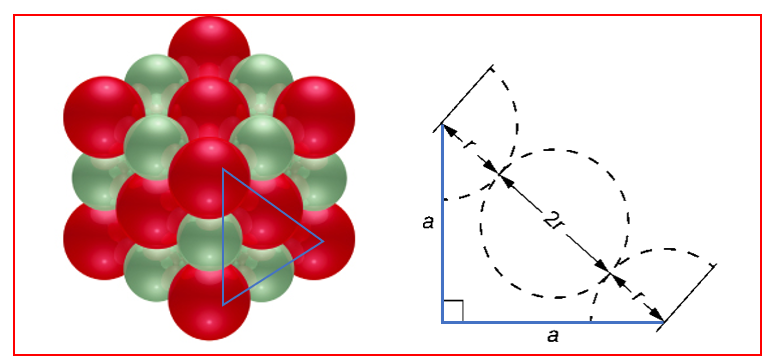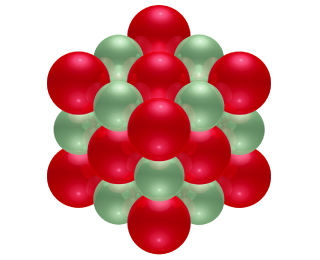# Problem: The ionic substance strontium oxide, SrO, forms from the direct reaction of strontium metal with molecular oxygen. The arrangement of the ions in solid SrO is analogous to that in solid NaCl and is shown here. Based on the ionic radii r(O2-) = 1.40 Å and r(Sr2+) = 1.13 Å, predict the length of the side of the cube in the figure.

###### FREE Expert Solution

We’re being asked to determine the edge length of the side of a cube for Strontium oxide, SrO knowing that its ions are arranged similar to that in NaCl and the given the ionic radius of Sr2+ and O2-.

Recall that the face-centered cubic lattice (FCC) contains an atom in each of the face of the cube and an atom in each of the corners

Based on the given diagram, SrO shows a face-centered cubic lattice (FCC).

On the face of a SrO unit cell, oxide ions contact each other across the diagonal of the face:81% (441 ratings)###### Problem Details

The ionic substance strontium oxide, SrO, forms from the direct reaction of strontium metal with molecular oxygen. The arrangement of the ions in solid SrO is analogous to that in solid NaCl and is shown here.Based on the ionic radii r(O2-) = 1.40 Å and r(Sr2+) = 1.13 Å, predict the length of the side of the cube in the figure.